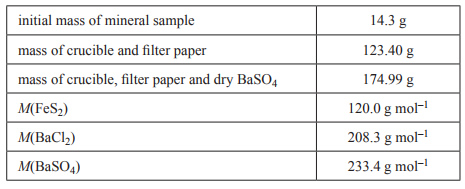Gravimetric analysis (2016 VCE) 1) A common iron ore, fool’s gold, contains the mineral iron pyrite, FeS2. Typically, the percentage by mass of FeS2 in a sample of fool’s gold is between 90% and 95%. The actual percentage in a sample can be determined by gravimetric analysis. The sulfur in FeS2 is converted to sulfate ions, SO42–. This is then mixed with an excess of barium chloride, BaCl2, to form barium sulfate, BaSO4, according to the equation Ba2+(aq) + SO42–(aq) → BaSO4s) When the reaction has gone to completion, the BaSO4 precipitate is collected in a filter paper and carefully washed. The filter paper and its contents are then transferred to a crucible. The crucible and its contents are heated until constant mass is achieved. The data for an analysis of a mineral sample is as follows.Calculate the percentage by mass of FeS2 in this mineral sample and state one assumption that was made in calculating the result.. Solution